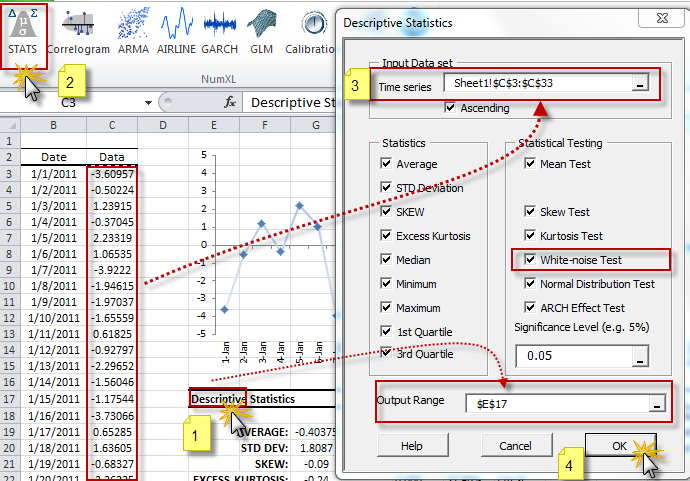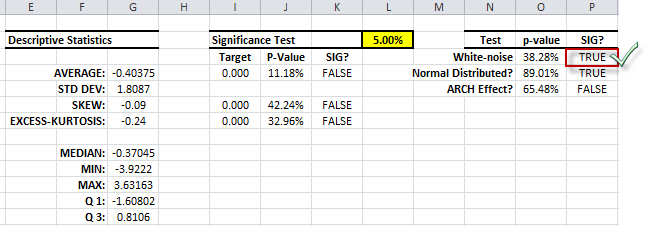# How do I test whether a given time series is just white‐noise?

In a statistical sense, a time series ${x_t}$ is characterized as having a weak white test in Excel (white noise) if ${x_t}$ is a sequence of serially uncorrelated random variables with zero mean and finite variance. Strong white noise also has the quality of being independent and identically distributed, which implies no autocorrelation.

In particular, if $x_t$ is normally distributed with mean zero and standard deviation $\sigma$, the series is called a Gaussian white noise (white test in Excel).

## Statistical Testing

To examine the data series for evidence of any serial correlation, we use Ljung‐Box statistical test and the modified $Q^*(m)$ statistics.

The Ljung‐Box Test:

$$H_o:\rho_1=\rho_2=\rho_3=...=\rho_m=0$$ $$H_1:\exists \rho_{1\leqslant k\leq m} \neq 0$$

Where:

• $H_o$: Null Hypothesis (White noise)
• $H_1$: Alternative hypothesis (not white noise)
• $m$: Upper lag limit of the test. The upper lag limit can either be set by us or left for the function to pick a proper limit. Practitioners use $\ln{T}$ as a proper value for $m$.

## Ljung‐Box Statistics

The Ljung‐Box statistics (i.e. $Q^*(m)$) is an enhancement on the classical $\chi^2$ test especially for small samples size.

$$Q^*(m)=T(T+2)\sum_{j=1}^m{\frac{\rho_j^2}{T-j}} Q^*(m)\sim \chi_{\nu=m}^2$$

This is a one‐tail statistical test, A p‐value greater than the significance level ($\alpha$) leads us to not‐to‐reject the null hypothesis or, in other words, the time series is a white test in Excel (white noise).

## WNTest

WNTest function in NumXL computes the P‐value for the $Q^*(m)$ statistics for our sample data. The upper lag limit ($m$) is set by default to $\ln{T}$, but the user can override this value by passing a value for this parameter

Example: Using the NumXL descriptive statistics form, we computed the various summary statistics and performed Ljung-Box white noise test (WNTest) among others.The summary statistics and tests:The P-Value of the Ljung-Box white noise test (white test in Excel) is greater than significance level (i.e. $\alpha$), so we don't reject the white noise hypothesis ($H_o$), or, simply stated; there is no statistical evidence of a serial correlation, so the data can be white noise.